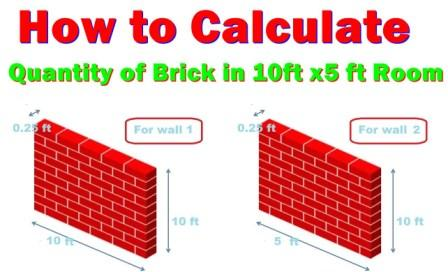How to calculate the number of bricks in 1 CFT wall

# How to calculate the number of bricks in 1 CFT wallHi, Civil Engineers, You might have knowledge about the quantity of bricks in one cubic meter wall. You may have question what is the number of brick in  1 cft wall so this articles  is important for you.
In this article I have explained about how to calculate the quantity of bricks in 1 CFT wall.
Before going through this article I recommend you to Know how to calculate the quantity cement sand aggregate and water for concrete Slab. Here I have taken the field size bricks

## Quantity of Bricks in 1 CFT Wall

Length of Bricks in cft (L) = 9” = 0.75 ft
Width of Bricks in cft (W) = 5” = 0.41 ft
Thickness of Bricks in cft (T) =3” = 0.25 ft
Volume of Bricks in cft = L x W x T
= 0.75 x 0.41 x 0.25
= 0.076 ft3
Numbers of bricks in 1 cft = 1/(0.076) =13.15 bricks
Mortar covered 10 % space so
=13.15 x (10/100)
=1.315
Quantity of bricks = 13.15 - 1.315 =11.835 bricks
Adding the 5 % wastage of Bricks in Quantity of bricks = 11.835 x(5/100) = 0.591

Hence, the total numbers of bricks in 1 cft =11.835 + 0.591 = 12.426
We can say 12.5 numbers of bricks required in 1 cft.

Do you want to know  how many numbers of Bricks required in Area of Room.

Let us use the above derivation by taking the room plan
Area of the room = 10 ft x 5 ft

## For Wall ( 1)

Length of one side wall = 10 ft
Width of bricks = 0.25 ft
Height of the wall = 10 ft
Volume of wall in cft = 10 x 0.25 x 10 =25 ft3
For both side= 2 x 25 = 50 ft3 (for wall 1)

Length of another wall = 5 ft
Width of bricks = 0.25 ft
Height of the wall = 10 ft
Volume of wall in cft = 5 x 0.25 x 10 = 12.5 ft3
For both sides = 2 x 12.5 = 25 ft3   ( for wall 2)

So total Volume of wall = Wall 1 + Wall 2
= 50 ft3 + 25 ft3   = 75 ft3
As per Above Derivation 1 cft =  12 .5 bricks
For 75 cft = 12.5 x 75 = 937.5 bricks required for making 10 ft x 5 ft  room.